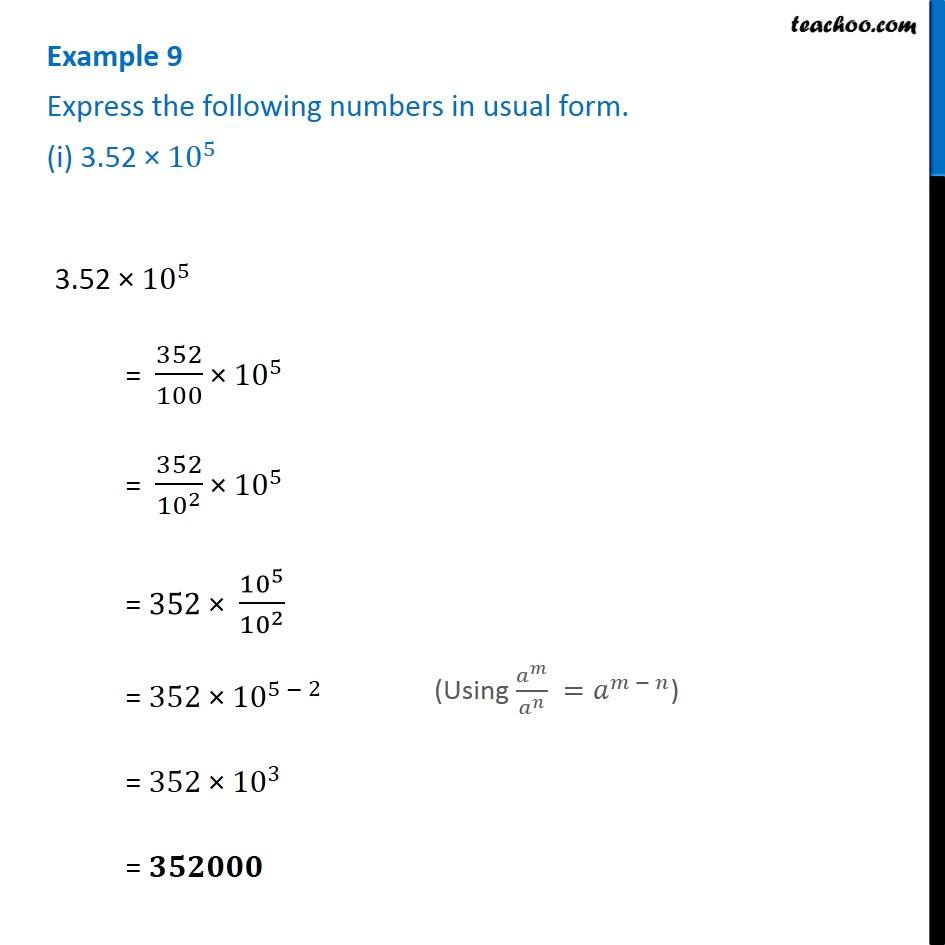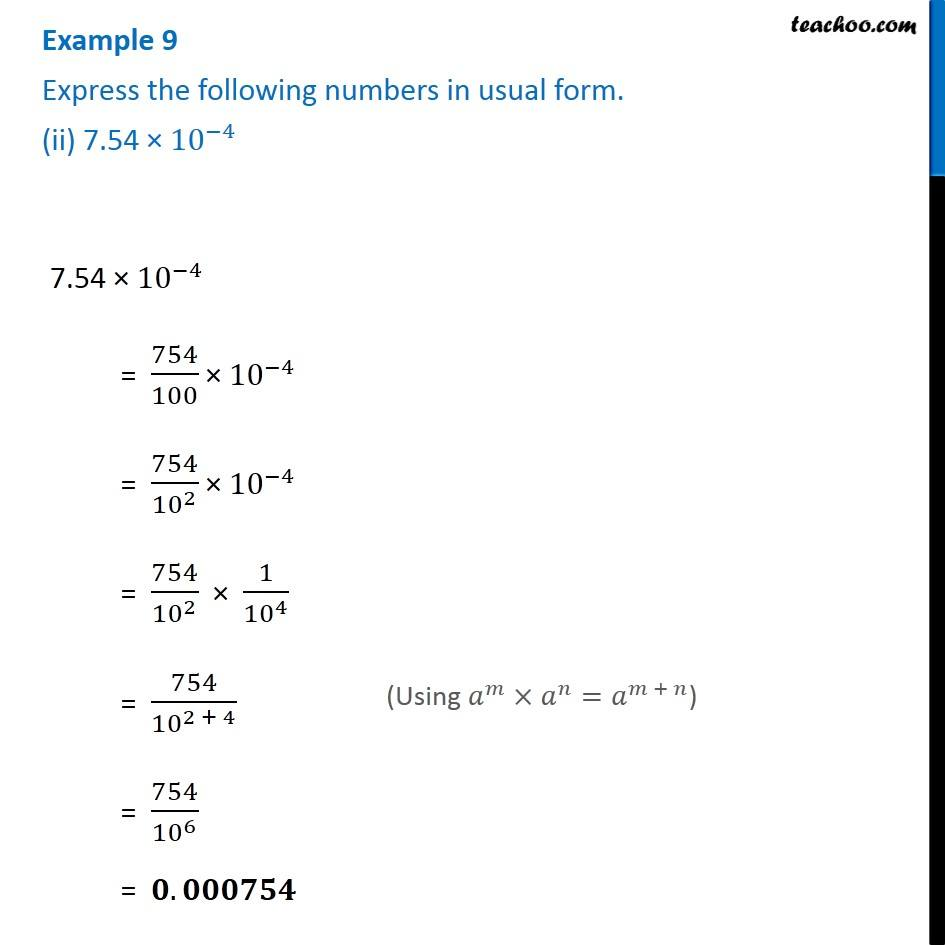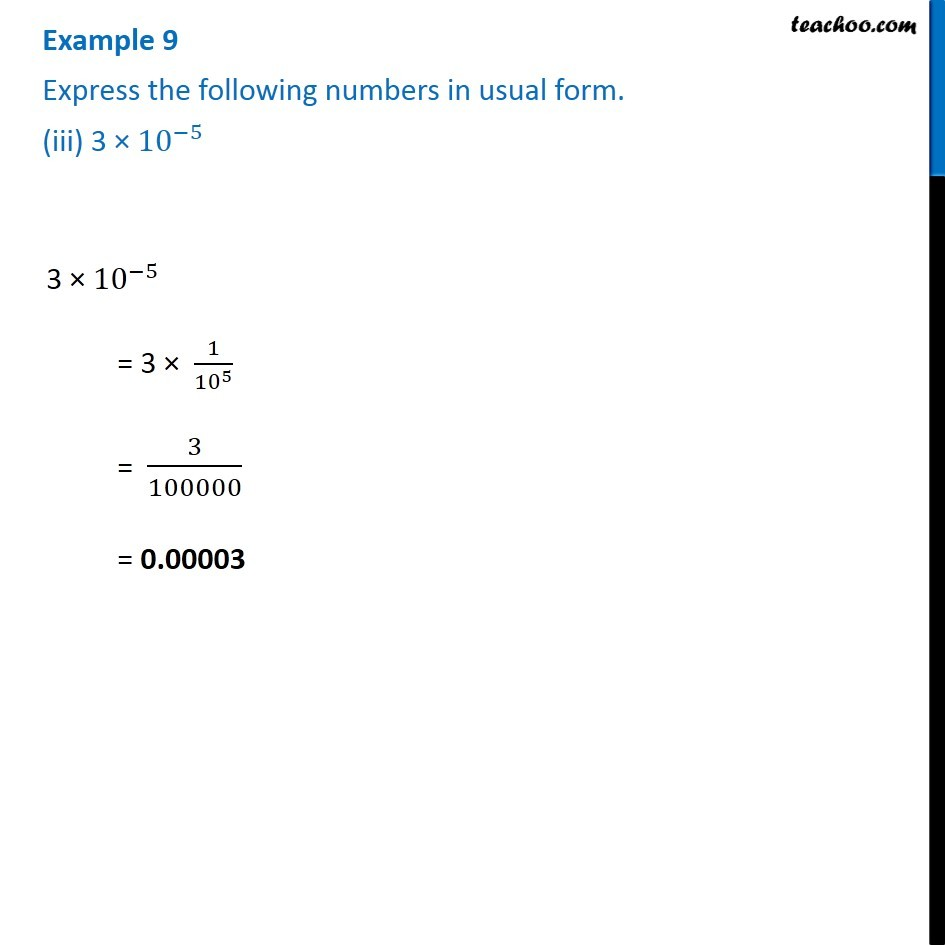1. Chapter 12 Class 8 Exponents and Powers
2. Serial order wise
3. Examples

Transcript

Example 9 Express the following numbers in usual form. (i) 3.52 × 〖10〗^5 3.52 × 〖10〗^5 = 352/100 × 〖10〗^5 = 352/〖10〗^2 × 〖10〗^5 = 352 × 〖10〗^5/〖10〗^2 = 352 × 〖10〗^(5 − 2) = 352 × 〖10〗^3 = 𝟑𝟓𝟐𝟎𝟎𝟎 (Using 𝑎^𝑚/𝑎^𝑛 =𝑎^(𝑚 − 𝑛)) Example 9 Express the following numbers in usual form. (ii) 7.54 × 〖10〗^(−4) 7.54 × 〖10〗^(−4) = 754/100 × 〖10〗^(−4) = 754/〖10〗^2 × 〖10〗^(−4) = 754/〖10〗^2 × 1/〖10〗^4 = 754/〖10〗^(2 + 4) = 754/〖10〗^6 = 𝟎.𝟎𝟎𝟎𝟕𝟓𝟒 (Using 𝑎^𝑚×𝑎^𝑛=𝑎^(𝑚 + 𝑛)) Example 9 Express the following numbers in usual form. (iii) 3 × 〖10〗^(−5) 3 × 〖10〗^(−5) = 3 × 1/〖10〗^5 = 3/100000 = 0.00003

Examples

About the AuthorDavneet Singh
Davneet Singh is a graduate from Indian Institute of Technology, Kanpur. He has been teaching from the past 10 years. He provides courses for Maths and Science at Teachoo.